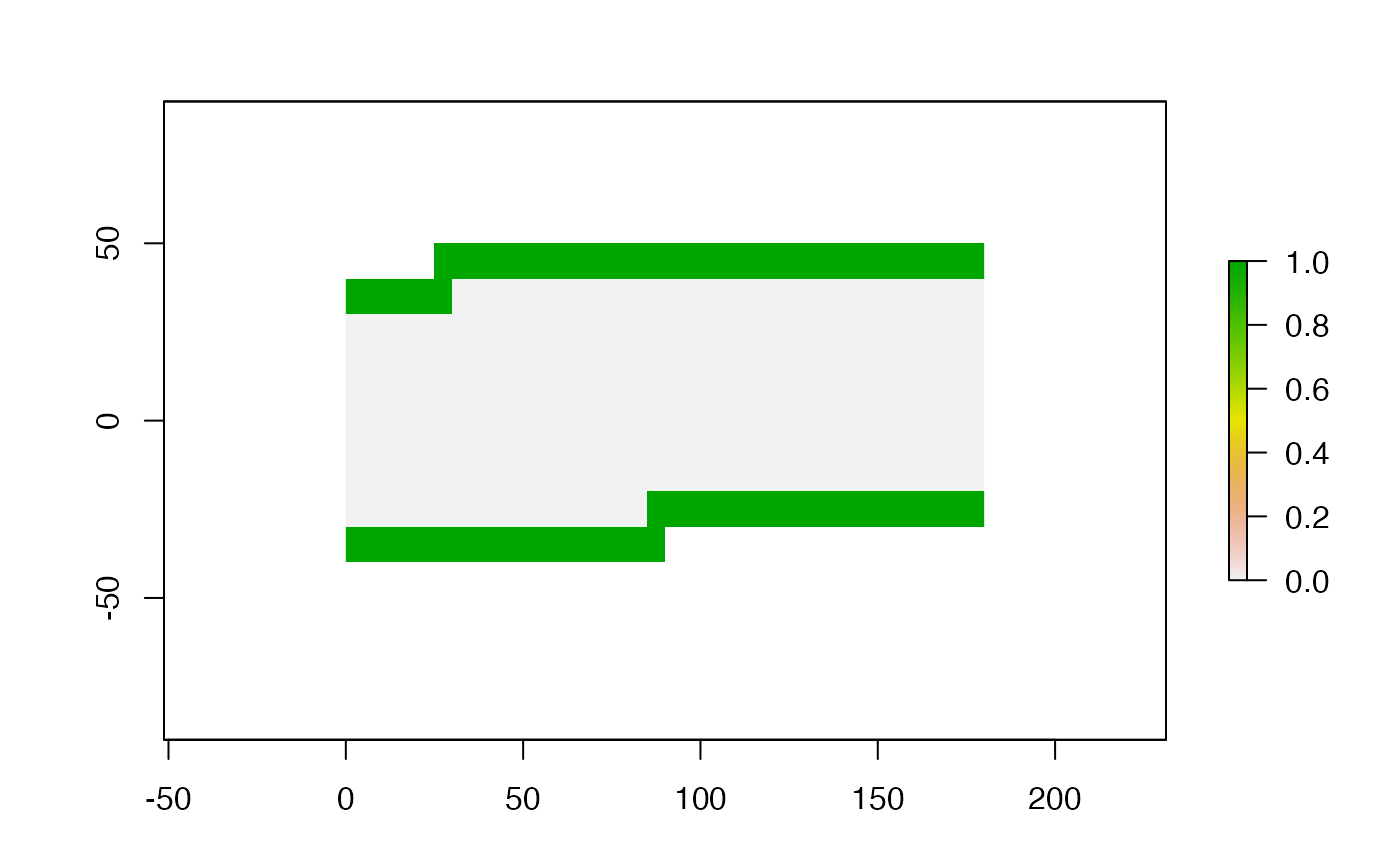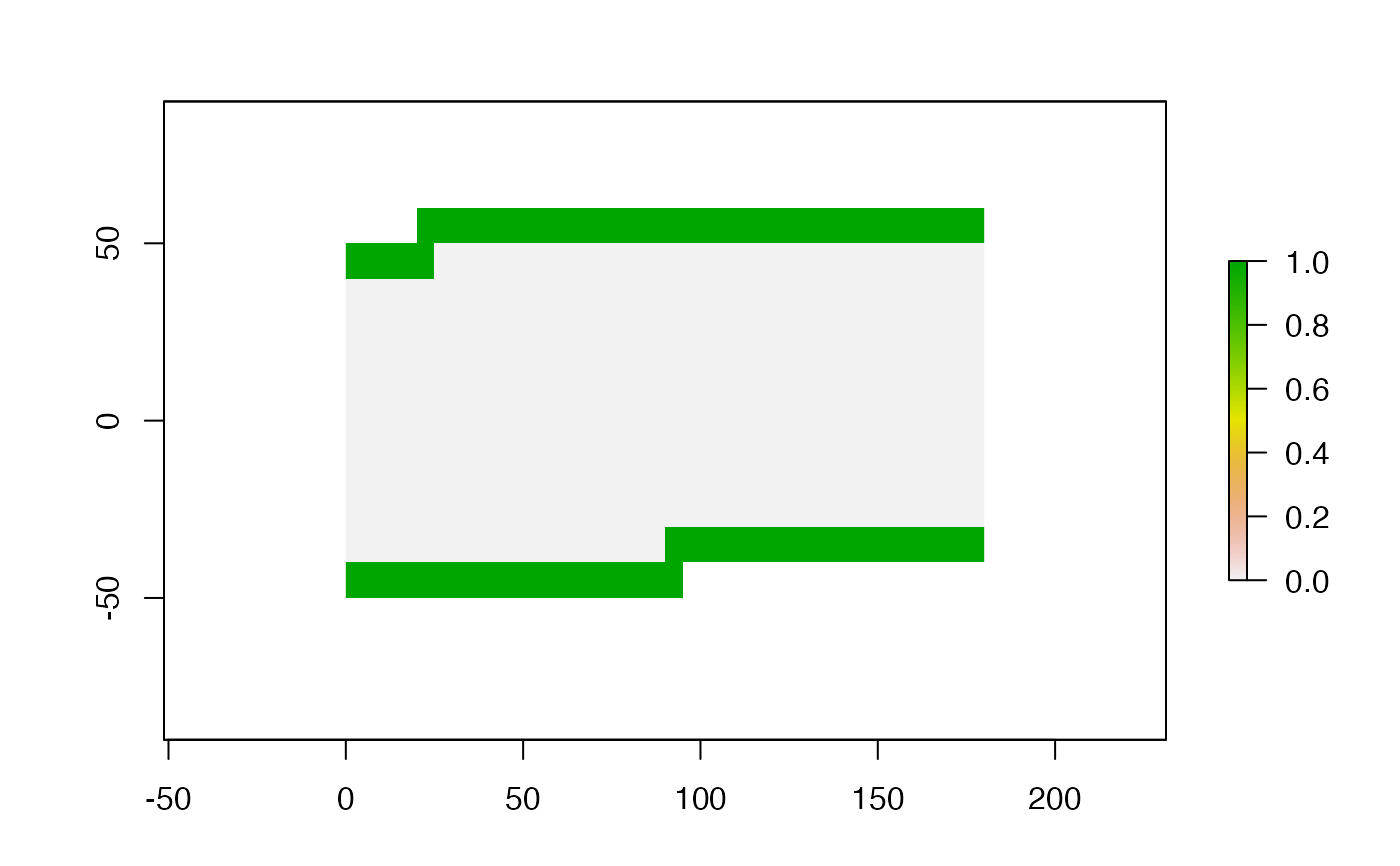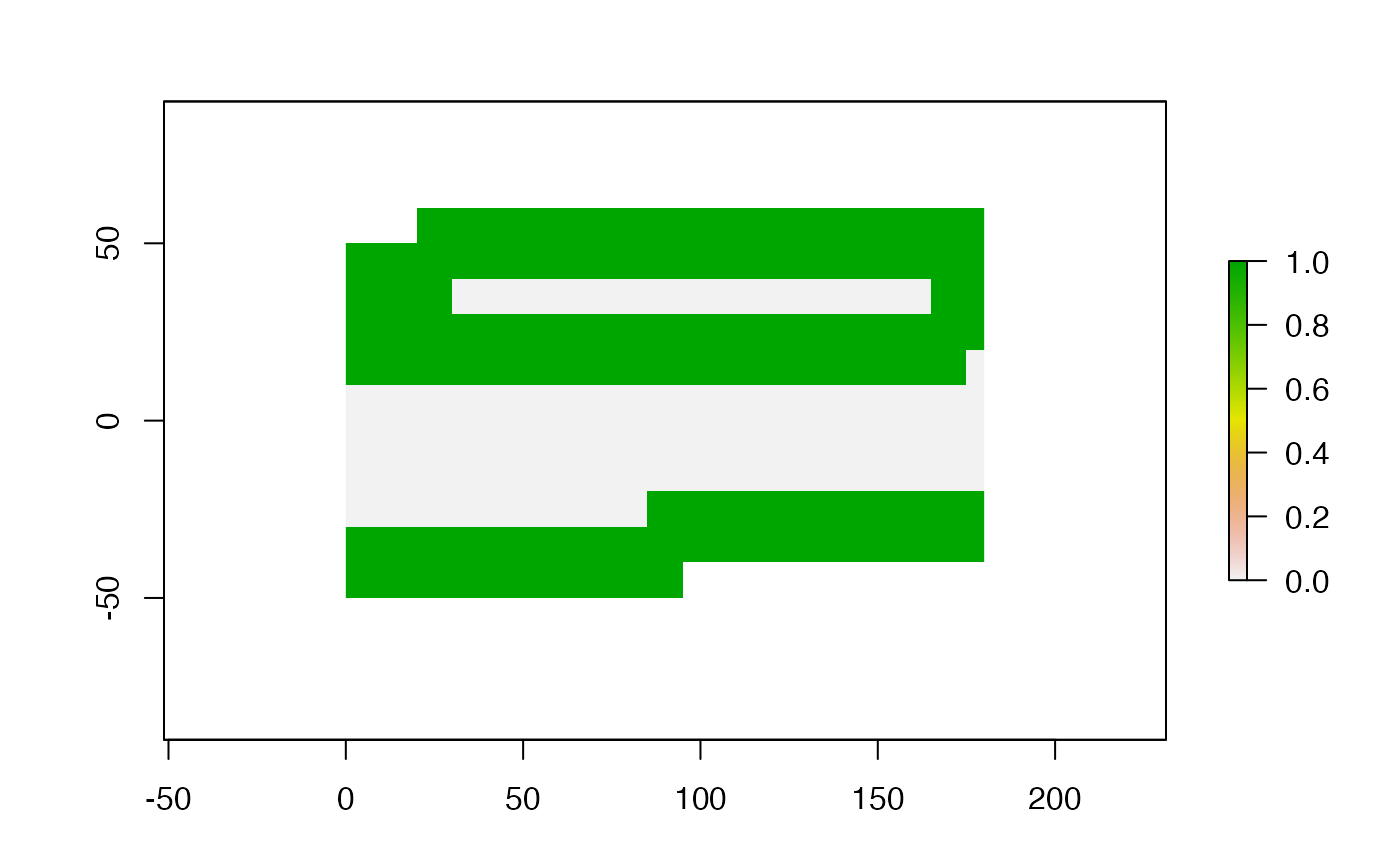Detect boundaries (edges). boundaries are cells that have more than one class in the 4 or 8 cells surrounding it, or, if classes=FALSE, cells with values and cells with NA.

# S4 method for RasterLayer
boundaries(x, type='inner', classes=FALSE, directions=8, asNA=FALSE, filename="", ...)

## Arguments

x

RasterLayer object

type

character. 'inner' or 'outer'

classes

character. Logical. If TRUE all different values are (after rounding) distinguished, as well as NA. If FALSE (the default) only edges between NA and non-NA cells are considered

directions

integer. Which cells are considered adjacent? Should be 8 (Queen's case) or 4 (Rook's case)

asNA

logical. If TRUE, non-edges are returned as NA instead of zero

filename

character. Filename for the output RasterLayer (optional)

...

additional arguments as for writeRaster

## Value

RasterLayer. Cell values are either 1 (a border) or 0 (not a border), or NA

focal, clump

## Examples

r <- raster(nrow=18, ncol=36, xmn=0)
r[150:250] <- 1
r[251:450] <- 2
plot( boundaries(r, type='inner') )plot( boundaries(r, type='outer') )plot( boundaries(r, classes=TRUE) )